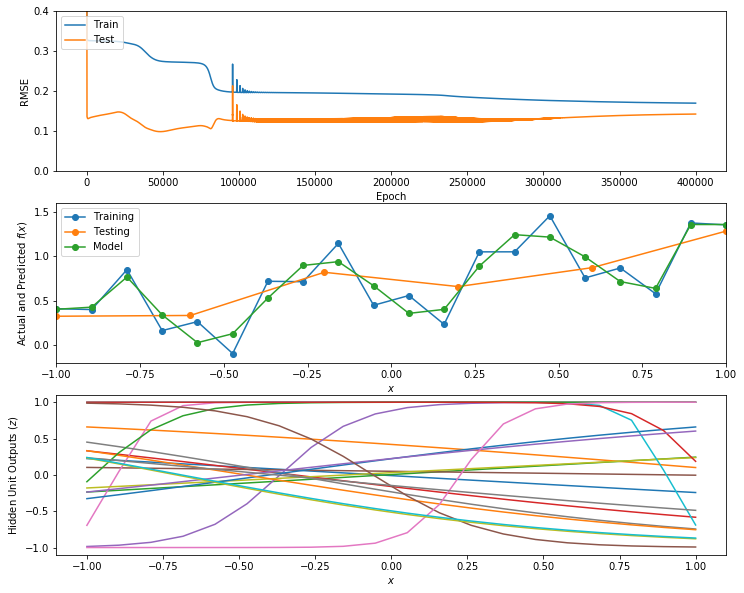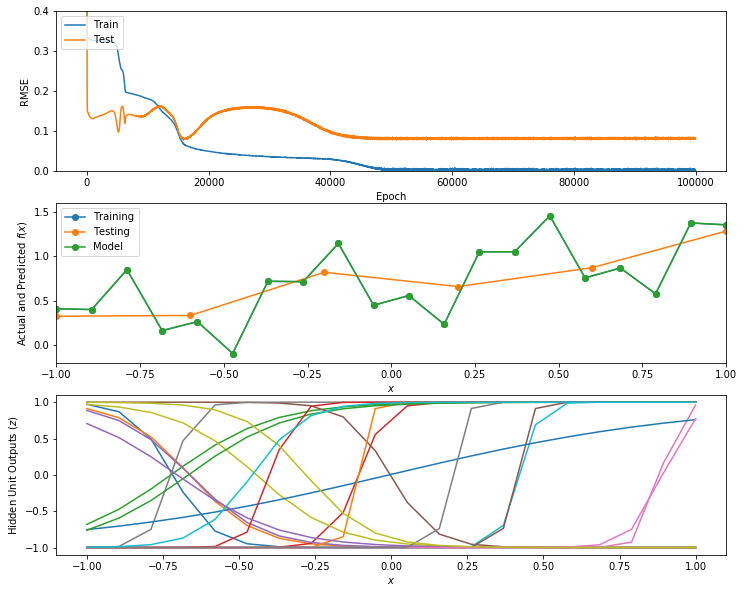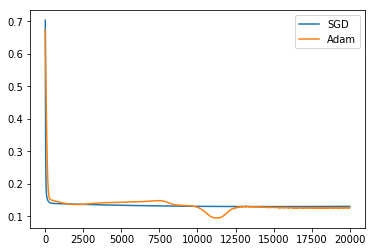$\newcommand{\xv}{\mathbf{x}} \newcommand{\Xv}{\mathbf{X}} \newcommand{\yv}{\mathbf{y}} \newcommand{\Yv}{\mathbf{Y}} \newcommand{\zv}{\mathbf{z}} \newcommand{\av}{\mathbf{a}} \newcommand{\Wv}{\mathbf{W}} \newcommand{\wv}{\mathbf{w}} \newcommand{\gv}{\mathbf{g}} \newcommand{\Hv}{\mathbf{H}} \newcommand{\dv}{\mathbf{d}} \newcommand{\Vv}{\mathbf{V}} \newcommand{\vv}{\mathbf{v}} \newcommand{\tv}{\mathbf{t}} \newcommand{\Tv}{\mathbf{T}} \newcommand{\zv}{\mathbf{z}} \newcommand{\Zv}{\mathbf{Z}} \newcommand{\muv}{\boldsymbol{\mu}} \newcommand{\sigmav}{\boldsymbol{\sigma}} \newcommand{\phiv}{\boldsymbol{\phi}} \newcommand{\Phiv}{\boldsymbol{\Phi}} \newcommand{\Sigmav}{\boldsymbol{\Sigma}} \newcommand{\Lambdav}{\boldsymbol{\Lambda}} \newcommand{\half}{\frac{1}{2}} \newcommand{\argmax}{\underset{#1}{\operatorname{argmax}}} \newcommand{\argmin}{\underset{#1}{\operatorname{argmin}}} \newcommand{\dimensionbar}{\underset{#1}{\operatorname{|}}}$

# A2 Adam vs SGD¶

Type your name here.

In :
import numpy as np
import matplotlib.pyplot as plt
%matplotlib inline

import IPython.display as ipd  # for display and clear_output
import time  # for sleep


Let's create a long vector of all of the weights in both layers. Then we can define the weight matrix for each layer as views into this vector. This allows us to take steps down the gradient of the error function by incrementing the whole weight vector.

Spend a little time understanding the difference between numpy views and copies. Here is a good tutorial.

In :
def make_weights(shapes):
'''make_weights(shape): weights is list of pairs of (n_inputs, n_units) for each layer.
n_inputs includes the constant 1 input.
Returns weight vector w of all weights, and list of matrix views into w for each layer'''
# Make list of number of weights in each layer
n_weights_each_matrix = [sh * sh for sh in shapes]
# Total number of weights
n_weights = sum(n_weights_each_matrix)
# Allocate weight vector with component for each weight
w = np.zeros(n_weights)
# List Ws will be list of weight matrix views into w for each layer
w_views = make_views_on_weights(w, shapes)
return w, w_views

In :
def make_views_on_weights(w, shapes):
w_views = []
first = 0
for sh in shapes:
# Create new view of w[first:last]
last = first + sh * sh
# Create new view of w[first:last] as matrix W to be matrix for a layer
W = w[first:last].reshape(sh)
# Initialize weight values to small uniformly-distributed values
n_inputs = sh
scale = 1.0 / np.sqrt(n_inputs)
W[:] = np.random.uniform(-scale, scale, size=sh)
# Add to list of W matrices, Ws.
w_views.append(W)
first = last
return w_views

In :
# Set parameters of neural network
nHiddens = 10
nOutputs = 1

# Initialize weights to uniformly distributed values between small normally-distributed between -0.1 and 0.1
Vshape = (1 +1, nHiddens)
Wshape = (nHiddens + 1, nOutputs)
w, [V, W] = make_weights([Vshape, Wshape])

print('w\n', w)
print('V\n', V)
print('W\n', W)

w
[ 0.50008358 -0.41142396 -0.68792698 -0.0087392   0.46763318  0.52808727
-0.49390623 -0.27083857  0.64929521  0.42054484  0.01881059  0.14739445
-0.21581996  0.63881736 -0.44896168 -0.68442318  0.38783159 -0.355871
0.28255684  0.05992597 -0.05823583  0.09436599  0.19962223  0.07702639
-0.11914825  0.25964552  0.0935374  -0.20997033 -0.14268473 -0.16555897
-0.18868223]
V
[[ 0.50008358 -0.41142396 -0.68792698 -0.0087392   0.46763318  0.52808727
-0.49390623 -0.27083857  0.64929521  0.42054484]
[ 0.01881059  0.14739445 -0.21581996  0.63881736 -0.44896168 -0.68442318
0.38783159 -0.355871    0.28255684  0.05992597]]
W
[[-0.05823583]
[ 0.09436599]
[ 0.19962223]
[ 0.07702639]
[-0.11914825]
[ 0.25964552]
[ 0.0935374 ]
[-0.20997033]
[-0.14268473]
[-0.16555897]
[-0.18868223]]


Now for some functions for calculating the output of our network, and for backpropagating the error to get a gradient of error with respect to all weights.

In :
def forward(w_views, X1):
# Forward pass on training data
V, W = w_views
Z = np.tanh(X1 @ V)
Z1 = np.insert(Z, 0, 1, 1)
Y = Z1 @ W
return Z1, Y

In :
def backward(w_views, X1, Z1, T, error):
V, W = w_views
# Backward pass.
# Calculate the gradient of squared error with respect to all weights in w.
#   Order of in w is all hidden layer weights followed by all output layer weights,
#   so gradient values are ordered this way.
gradient =  np.hstack(((- X1.T @ ( ( error @ W[1:, :].T) * (1 - Z1[:, 1:]**2))).flat,  # for hidden layer
(- Z1.T @ error).flat))  # for output layer
return gradient


Given these functions, we can now define the stochastic gradient descent, sgd, procedure to update the weights.

In :
def sgd_init():
pass

def sgd(w, w_views, X1, T, learning_rate):
Z1, Y = forward(w_views, X1)

# Error in output
n_samples = X1.shape
n_outputs = T.shape

error = (T - Y) / (n_samples + n_outputs)

gradient = backward(w_views, X1, Z1, T, error)

# update values of w, in place. Don't need to return it.

w -= learning_rate * gradient


Here is another way to update the weights, the adam procedure. See this discussion of Adam and other gradient descent methods.

In :
grad_trace = 0
grad_squared_trace = 0
update_step = 0

def adam_init():
global grad_trace, grad_squared_trace, update_step

grad_trace = 0
grad_squared_trace = 0
update_step = 0

def adam(w, w_views, X1, T, learning_rate):
global grad_trace, grad_squared_trace, update_step

beta1 = 0.9
beta2 = 0.999
epsilon = 1e-8

Z1, Y = forward(w_views, X1)

# Error in output
error = T - Y

gradient = backward(w_views, X1, Z1, T, error)

# approximate first and second moment
grad_trace = beta1 * grad_trace + (1 - beta1) * gradient
grad_squared_trace = beta2 * grad_squared_trace + (1 - beta2) * np.square(gradient)

# bias corrected moment estimates
grad_hat = grad_trace / (1 - beta1 ** (update_step + 1) )
grad_squared_hat = grad_squared_trace / (1 - beta2 ** (update_step + 1) )

dw = grad_hat / (np.sqrt(grad_squared_hat) + epsilon)

n_samples = X1.shape
n_outputs = T.shape

# update values of w, in place. Don't need to return it.
w -= learning_rate / (n_samples + n_outputs) * dw

update_step += 1


Now, wrap these all together into a function to train a neural network given the training and testing data, the gradient descent function names, the batch size, the number of epochs, the learning rate, and the graphics update rate.

In :
def train(Xtrain, Ttrain, Xtest, Ttest,
n_hiddens,
gradient_descent_method_init, gradient_descent_method,
batch_size, n_epochs, learning_rate, graphics_rate=0):

if graphics_rate > 0 and Xtrain.shape > 1:
print('Graphics only works when X has one column (data has one input variable)')
print('Setting graphics_rate to 0')
graphics_rate = 0

# Initialize weights to uniformly distributed values between small normally-distributed between -0.1 and 0.1
n_inputs = Xtrain.shape
n_outputs = Ttrain.shape
Vshape = (1 + n_inputs, n_hiddens)
Wshape = (1 + n_hiddens, n_outputs)
w, [V, W] = make_weights([Vshape, Wshape])

error_trace = np.zeros((n_epochs, 2))

if graphics_rate > 0:
fig = plt.figure(figsize=(12, 10))

Xtrain1 = np.insert(Xtrain, 0, 1, 1)
Xtest1 = np.insert(Xtest, 0, 1, 1)
n_samples = Xtrain1.shape

gradient_descent_method_init()

for epoch in range(n_epochs):

# gradient_descent_method_init()

# Reorder samples
rows = np.arange(n_samples)
np.random.shuffle(rows)

for first_n in range(0, n_samples, batch_size):
last_n = first_n + batch_size
rows_batch = rows[first_n:last_n]
Xtrain1_batch = Xtrain1[rows_batch, :]
Ttrain_batch = Ttrain[rows_batch, :]
# gradient_descent method changes values of w
gradient_descent_method(w, [V, W], Xtrain1_batch, Ttrain_batch, learning_rate)

# error traces for plotting
Z1train, Ytrain = forward([V, W], Xtrain1)
error_trace[epoch, 0] = np.sqrt(np.mean(((Ttrain - Ytrain)**2)))

Z1test, Ytest = forward([V, W], Xtest1)
error_trace[epoch, 1] = np.sqrt(np.mean((Ytest - Ttest)**2))

if graphics_rate > 0 and (epoch % graphics_rate == 0 or epoch == n_epochs - 1):
plt.clf()
plt.subplot(3, 1, 1)
plt.plot(error_trace[:epoch, :])
plt.ylim(0, 0.4)
plt.xlabel('Epoch')
plt.ylabel('RMSE')
plt.legend(('Train','Test'), loc='upper left')

plt.subplot(3, 1, 2)
plt.plot(Xtrain, Ttrain, 'o-', Xtest, Ttest, 'o-', Xtrain, Ytrain, 'o-')
plt.xlim(-1, 1)
plt.ylim(-0.2, 1.6)
plt.legend(('Training', 'Testing', 'Model'), loc='upper left')
plt.xlabel('$x$')
plt.ylabel('Actual and Predicted $f(x)$')

plt.subplot(3, 1, 3)
plt.plot(Xtrain, Z1train[:, 1:])  # Don't plot the constant 1 column
plt.ylim(-1.1, 1.1)
plt.xlabel('$x$')
plt.ylabel('Hidden Unit Outputs ($z$)');

ipd.clear_output(wait=True)
ipd.display(fig)

ipd.clear_output(wait=True)

return Ytrain, Ytest, error_trace


Here are some demonstrations.

In :
# Make some training data
n = 20
Xtrain = np.linspace(0.,20.0,n).reshape((n,1)) - 10
Ttrain = 0.2 + 0.05 * (Xtrain + 10) + 0.4 * np.sin(Xtrain + 10) + 0.2 * np.sin(Xtrain * 3) + 0.01 * np.random.normal(size=(n, 1))
Xtrain = Xtrain / 10

# Make some testing data
n = n // 3
Xtest = np.linspace(0, 20, n).reshape((-1, 1)) - 10
Ttest = 0.2 + 0.05 * (Xtest + 10) + 0.2 * np.sin(Xtest + 10) +  0.1 * np.sin(Xtest * 3) + 0.01 * np.random.normal(size=(n, 1))
Xtest = Xtest / 10

In :
Ytrain, Ytest, error_trace = train(Xtrain, Ttrain, Xtest, Ttest, n_hiddens=20,
gradient_descent_method_init=sgd_init, gradient_descent_method=sgd,
batch_size=Xtrain.shape, n_epochs=400000, learning_rate=0.2, graphics_rate=10000)
print('Final RMSE', np.sqrt(np.mean((Ttest - Ytest)**2)))

Final RMSE 0.14267154975279336In :
Ytrain, Ytest, error_trace = train(Xtrain, Ttrain, Xtest, Ttest, n_hiddens=20,
gradient_descent_method_init=adam_init, gradient_descent_method=adam,
batch_size=Xtrain.shape, n_epochs=100000, learning_rate=0.05, graphics_rate=5000)
print('Final RMSE', np.sqrt(np.mean((Ttest - Ytest)**2)))

Final RMSE 0.0817539592336979In :
n_samples = Xtrain.shape

_, _, error_trace_adam = train(Xtrain, Ttrain, Xtest, Ttest, n_hiddens=20,
gradient_descent_method_init=adam_init, gradient_descent_method=adam,
batch_size=n_samples, n_epochs=20000, learning_rate=0.01, graphics_rate=0)

_, _, error_trace_sgd = train(Xtrain, Ttrain, Xtest, Ttest, n_hiddens=20,
gradient_descent_method_init=sgd_init, gradient_descent_method=sgd,
batch_size=n_samples, n_epochs=20000, learning_rate=0.01, graphics_rate=0)

plt.plot(np.hstack((error_trace_sgd[:, 1:], error_trace_adam[:, 1:])))
plt.legend(('SGD', 'Adam'))

print('SGD', error_trace_sgd[-1, 1], 'Adam', error_trace_adam[-1, 1])

SGD 0.13034848694643364 Adam 0.1259439754844665# Search for Good Parameter Values on a New Data Set¶

Now your work begins. First, download this Real Estate Valuation Data from the UCI machine learning repository. Read it in to python and form an input matrix X that contains six columns, and target matrix T of one column containing the house price of unit area. This is easiest to do with the pandas package. Check out the Getting Started material at the pandas site. Near the bottom of that page are some simple examples of how to use the read_excel pandas function. Pretty handy since the Real Estate data is an .xlsx file. Other helpful functions are the drop function that can be used to remove a column, such as the one labeled No in the data file, which is just an index that we should ignore, and data.columns.tolist() where data is a Dataframe. Also note that a Dataframe can be converted to a numpy array by npdata = np.array(data) where data again is a Dataframe.

We want to try to predict the target value from the six input values.

Randomly partition the data into an 80% partition for training, making Xtrain and Ttrain, and a 20% partition for testing, makng Xtest and Ttest.

Standardize the input Xtrain and Xtest matrices by subtracting by the column means and dividing by the column standard deviations, with the means and standard deviations determined only from the Xtrain matrix.

Using the one-hidden layer neural network implemented here, train neural networks in two ways: one with SGD and one with Adam. For each, try at least three values for each of the following parameters:

• number of hidden units, from 1 to 50,
• batch size,
• number of epochs
• learning_rate

Create a table of results containing the algorithm name ('sgd' or 'adam'), the values of the above four parameters, and the RMSE on the training and testing data. Since this is a mixed-type table, use the pandas package. Sort your table by the test RMSE.

Here are some clues on how to do this. To initialize a pandas Dataframe, called results do

  import pandas as pd

results = pd.DataFrame(columns=['Algorithm', 'Epochs', 'Learning Rate', 'Hidden Units', 'Batch Size',
'RMSE Train', 'RMSE Test'])


To add a row to this, do

  results.loc[len(results)] = [algo, n_epochs, lr, nh, bs, rmse(Ytrain, Ttrain), rmse(Ytest, Ttest)]



assuming those variables have appropriate values. Then, to sort results by RMSE Test and just see the top 50 entries, do

  results.sort_values('RMSE Test').head(50)

Put the above steps into a new function named run_parameters that accepts the arguments

* Xtrain, standardized
* Ttrain
* Xtest, standardized
* Ttest
* list of numbers of epochs
* list of learning rates
* list of numbers of hidden units
* list of batch sizes
* verbose, if True then print results of each parameter value combination



and returns a pandas DataFrame containing the results of runs for all combinations of the above parameter values. The DataFrame must have columns titled [Algorithm', 'Epochs', 'Learning Rate', 'Hidden Units', 'Batch Size', 'RMSE Train', 'RMSE Test']. So, if eac of the above lists contains two values, the resulting DataFrame must have 16 rows.

Describe your experiments, including how you decided on what values to test, and what the results tell you.

Extract the RMSE test values for all values of Hidden Units using the best values for the other parameters. Here is an example of how to do this.

nh =results.loc[(results['Algorithm'] == 'adam') & (results['Epochs'] == 100) &
(results['Learning Rate'] == 0.002) & (results['Batch Size'] == 1)]



Now you can plot the training and testing RMSE versus the hidden units. Describe what you see.

## Grading¶

Download A2grader.tar and extract A2grader.py from it. Run the code in the following cell to demonstrate an example grading session. You should see a perfect execution score of 90 out of 90 points if your functions are defined correctly. The remaining 10 points will be based on the results you obtain from the energy data and on your discussions.

For the grading script to run correctly, you must first name this notebook as 'Lastname-A2.ipynb' with 'Lastname' being your last name, and then save this notebook.

A different, but similar, grading script will be used to grade your checked-in notebook. It will include additional tests. You need not include code to test that the values passed in to your functions are the correct form.

In :
%run -i A2grader.py

======================= Code Execution =======================

Extracting python code from notebook named 'ANSWERS A2 Adam vs SGD -A2.ipynb' and storing in notebookcode.py
Removing all statements that are not function or class defs or import statements.
Testing:
data = np.loadtxt('machine.data', delimiter=',', usecols=range(2, 10))
X = data[:, :-2]
T = data[:, -2:-1]
Xtrain = X[:160, :]
Ttrain = T[:160, :]
Xtest = X[160:, :]
Ttest = T[160:, :]

means = Xtrain.mean(0)
stds = Xtrain.std(0)
Xtrains = (Xtrain - means) / stds
Xtests = (Xtest - means) / stds

results = run_parameters(Xtrains, Ttrain, Xtests, Ttest, [10, 100], [0.001, 0.01], [5, 10], [1, 50])

Algorithm Epochs Learning Rate Hidden Units Batch Size RMSE Train RMSE Test
0 adam 10 0.001 5 1 171.289852 243.774031
1 adam 10 0.001 5 50 172.770654 245.297324
2 adam 10 0.001 10 1 170.534144 242.986122
3 adam 10 0.001 10 50 172.582129 244.938442
4 adam 10 0.010 5 1 159.639379 233.124807
5 adam 10 0.010 5 50 172.358288 244.812005
6 adam 10 0.010 10 1 151.848659 225.831455
7 adam 10 0.010 10 50 172.491806 245.067770
8 adam 100 0.001 5 1 159.543102 233.044486
9 adam 100 0.001 5 50 172.574566 244.916769
10 adam 100 0.001 10 1 152.212294 226.171767
11 adam 100 0.001 10 50 172.587105 244.972892
12 adam 100 0.010 5 1 112.784060 187.344367
13 adam 100 0.010 5 50 171.907782 244.322314
14 adam 100 0.010 10 1 92.788360 189.771543
15 adam 100 0.010 10 50 171.902010 244.288952
16 sgd 10 0.001 5 1 110.608169 184.531282
17 sgd 10 0.001 5 50 164.889235 237.815073
18 sgd 10 0.001 10 1 94.172841 176.651406
19 sgd 10 0.001 10 50 160.091022 233.179509
20 sgd 10 0.010 5 1 102.237991 177.489071
21 sgd 10 0.010 5 50 125.844944 215.791480
22 sgd 10 0.010 10 1 90.717217 195.537095
23 sgd 10 0.010 10 50 113.820425 216.468660
24 sgd 100 0.001 5 1 79.710451 161.974949
25 sgd 100 0.001 5 50 124.036346 198.349385
26 sgd 100 0.001 10 1 60.622768 145.282637
27 sgd 100 0.001 10 50 108.383455 186.720139
28 sgd 100 0.010 5 1 83.582095 167.189971
29 sgd 100 0.010 5 50 80.362801 176.195046
30 sgd 100 0.010 10 1 99.783754 187.778786
31 sgd 100 0.010 10 50 71.816796 161.769054
--- 40/40 points. run_parameters terminates.

--- 20/20 points. results.shape is correct.

--- 10/10 points. results column names is correct.

--- 10/10 points. Values in results Algorithm column correct.

--- 10/10 points. Number of epochs for lowest RMSE Train is correct.

A2 Execution Grade is 90 / 90

Remaining 10 points will be based on your text descriptions of results and plots.

A2 FINAL GRADE is   / 100

A2 EXTRA CREDIT is   / 1


# Extra Credit¶

Repeat the evaluation of parameter values for another data set of your choice.